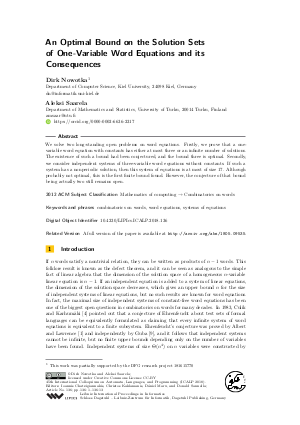Document# An Optimal Bound on the Solution Sets of One-Variable Word Equations and its Consequences

### Authors Dirk Nowotka, Aleksi Saarela## File

LIPIcs.ICALP.2018.136.pdf
• Filesize: 423 kB
• 13 pages

## Cite As

Dirk Nowotka and Aleksi Saarela. An Optimal Bound on the Solution Sets of One-Variable Word Equations and its Consequences. In 45th International Colloquium on Automata, Languages, and Programming (ICALP 2018). Leibniz International Proceedings in Informatics (LIPIcs), Volume 107, pp. 136:1-136:13, Schloss Dagstuhl - Leibniz-Zentrum für Informatik (2018)
https://doi.org/10.4230/LIPIcs.ICALP.2018.136

## Abstract

We solve two long-standing open problems on word equations. Firstly, we prove that a one-variable word equation with constants has either at most three or an infinite number of solutions. The existence of such a bound had been conjectured, and the bound three is optimal. Secondly, we consider independent systems of three-variable word equations without constants. If such a system has a nonperiodic solution, then this system of equations is at most of size 17. Although probably not optimal, this is the first finite bound found. However, the conjecture of that bound being actually two still remains open.

## Subject Classification

##### ACM Subject Classification
• Mathematics of computing → Combinatorics on words
##### Keywords
• combinatorics on words
• word equations
• systems of equations

## Metrics

• Access Statistics
• Total Accesses (updated on a weekly basis)
0

## References

1. M. H. Albert and J. Lawrence. A proof of Ehrenfeucht’s conjecture. Theoret. Comput. Sci., 41(1):121-123, 1985. URL: http://dx.doi.org/10.1016/0304-3975(85)90066-0.
2. Jean Berstel, Dominique Perrin, and Christophe Reutenauer. Codes and Automata. Cambridge University Press, 2010.3. L. G. Budkina and Al. A. Markov. F-semigroups with three generators. Mat. Zametki, 14:267-277, 1973.4. Karel Culik, II and Juhani Karhumäki. Systems of equations over a free monoid and Ehrenfeucht’s conjecture. Discrete Math., 43(2-3):139-153, 1983. URL: http://dx.doi.org/10.1016/0012-365X(83)90152-8.
5. Elena Czeizler and Juhani Karhumäki. On non-periodic solutions of independent systems of word equations over three unknowns. Internat. J. Found. Comput. Sci., 18(4):873-897, 2007. URL: http://dx.doi.org/10.1142/S0129054107005030.
6. Elena Czeizler and Wojciech Plandowski. On systems of word equations over three unknowns with at most six occurrences of one of the unknowns. Theoret. Comput. Sci., 410(30-32):2889-2909, 2009. URL: http://dx.doi.org/10.1016/j.tcs.2009.01.023.
7. Robert Da̧browski and Wojciech Plandowski. On word equations in one variable. Algorithmica, 60(4):819-828, 2011. URL: http://dx.doi.org/10.1007/s00453-009-9375-3.
8. S. Eyono Obono, P. Goralčík, and M. Maksimenko. Efficient solving of the word equations in one variable. In Proceedings of the 19th MFCS, volume 841 of LNCS, pages 336-341. Springer, 1994. URL: http://dx.doi.org/10.1007/3-540-58338-6_80.
9. V. S. Guba. Equivalence of infinite systems of equations in free groups and semigroups to finite subsystems. Mat. Zametki, 40(3):321-324, 1986. URL: http://dx.doi.org/10.1007/BF01142470.
10. Tero Harju and Dirk Nowotka. On the independence of equations in three variables. Theoret. Comput. Sci., 307(1):139-172, 2003. URL: http://dx.doi.org/10.1016/S0304-3975(03)00098-7.
11. Štěpán Holub and Jan Žemlička. Algebraic properties of word equations. J. Algebra, 434:283-301, 2015. URL: http://dx.doi.org/10.1016/j.jalgebra.2015.03.021.
12. Artur Jeż. One-variable word equations in linear time. Algorithmica, 74(1):1-48, 2016. URL: http://dx.doi.org/10.1007/s00453-014-9931-3.
13. Juhani Karhumäki and Wojciech Plandowski. On the defect effect of many identities in free semigroups. In Gheorghe Paun, editor, Mathematical aspects of natural and formal languages, pages 225-232. World Scientific, 1994.14. Juhani Karhumäki and Aleksi Saarela. On maximal chains of systems of word equations. Proc. Steklov Inst. Math., 274:116-123, 2011. URL: http://dx.doi.org/10.1134/S0081543811060083.
15. Markku Laine and Wojciech Plandowski. Word equations with one unknown. Internat. J. Found. Comput. Sci., 22(2):345-375, 2011. URL: http://dx.doi.org/10.1142/S0129054111008088.
16. Dirk Nowotka and Aleksi Saarela. One-variable word equations and three-variable constant-free word equations. Internat. J. Found. Comput. Sci., To appear.17. Aleksi Saarela. Systems of word equations, polynomials and linear algebra: A new approach. European J. Combin., 47:1-14, 2015. URL: http://dx.doi.org/10.1016/j.ejc.2015.01.005.
18. Aleksi Saarela. Word equations where a power equals a product of powers. In Proceedings of the 34th STACS, volume 66 of LIPIcs, pages 55:1-55:9. Schloss Dagstuhl-Leibniz-Zentrum fuer Informatik, 2017. URL: http://dx.doi.org/10.4230/LIPIcs.STACS.2017.55.
19. Jean-Claude Spehner. Quelques problémes d'extension, de conjugaison et de présentation des sous-monoïdes d'un monoïde libre. PhD thesis, Univ. Paris, 1976.20. Jean-Claude Spehner. Les systemes entiers d'équations sur un alphabet de 3 variables. In Semigroups, pages 342-357, 1986.X

Feedback for Dagstuhl Publishing